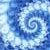Games
Problems
Go Pro!

# Digits

Pro Problems > Math > Number and Quantity > Number Theory > Digits

# Featured Pro ProblemsA math problem involving Shrek wanting Fiona's telephone numberGiven two pieces of information about the three digit number, determine all possible values.Determine a three digit number from sum and difference of reversed-digit numberFind how many two-digit numbers match the patterns specifiedDetermine my value, based on information about my digitsWhat happens when two three-digit numbers are placed back to back to create a six digit number?Figure out the digits of Rhonda's five-digit zip code using the clues givenFind the palindrome that lies in the middle of a listing of palindromes

# Full Directory Listing

All My Digits, Back to Back, Coffee Math, Digits in a Multiplication Problem, Fill in the blanks, Find the Number, Fiona's Telephone Number, Five Digit Number, Four Digit Number, Grapes on the Vine, Happy New Year, I Have Three Digits, My Three Digits, Palindrome Addition, Reverse Me, Rhonda's Zip Code, Set of Five Digit Numbers, Sum of Digits, The Middle Palindrome, Three Digit Difference, Three Digit Number, Three Digit Number, Three Digits with Difference, Three Digits, sum and product, Two Digit Pattern MatchingLike us on Facebook to get updates about new resources